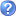• Free Math Help
Lessons, tutoring, message board and more. Algebra, Geometry, Trig, Calculus... whatever level you're studying!
Math lessons, tutoring, books, calculators and more. Finish your homework in no time with our free problem solvers and graphing tools. Extensive lesson library covering algebra, geometry, trig, and calculus. Get expert math help if you can't figure it out.
algebra geometry homework math school tutoring
by 3 users